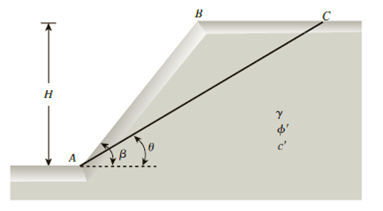Chapter 15, Problem 15.7PPrinciples of Geotechnical Enginee...

9th Edition
Braja M. Das + 1 other
ISBN: 9781305970939

Solutions

Chapter
SectionPrinciples of Geotechnical Enginee...

9th Edition
Braja M. Das + 1 other
ISBN: 9781305970939
Textbook Problem

Figure 15.49 shows a slope with an inclination of β = 58°. If AC represents a trial failure plane inclined at an angle θ = 32° with the horizontal, determine the factor of safety against sliding for the wedge ABC. Given: H = 6 m; γ = 19 kN/m3, ϕ ′ = 21 ° , and c′ = 38 kN/m2.Figure 15.49

To determine

Find the factor of safety Fs against sliding for the wedge ABC.

Explanation

Given information:

The vertical height (H) of the soil is 6.0 m.

The slope with an inclination β is 58°.

The failure plane inclined at an angle θ of 32°.

The angle of friction ϕ is 21°.

The cohesion c is 38kN/m2.

The unit weight γ of the soil is 19kN/m3.

Calculation:

Draw the free body diagram of the wedge ABC as in Figure 1.

Determine the weight W of the wedge ABC using the formula.

W=12γH2[sin(βθ)sinβsinθ]

Substitute 19kN/m3 for γ, 6.0 m for H, 58° for β, and 32° for θ.

W=12(19)(6.0)2[sin(58°32°)sin58°sin32°]=333.6kN

Determine the normal component Ta using the relation.

Ta=Wsinθ

Substitute 333.6 kN for W and 32° for θ.

Ta=333.6sin32°=176.78kN

Determine the tangential component Na using the relation.

Na=Wcosθ

Substitute 333.6 kN for W and 32° for θ

Still sussing out bartleby?

Check out a sample textbook solution.

See a sample solution

The Solution to Your Study Problems

Bartleby provides explanations to thousands of textbook problems written by our experts, many with advanced degrees!

Get Started

16.28 Problem 16.28

Engineering Fundamentals: An Introduction to Engineering (MindTap Course List)

Which security protocols are used to protect e-mail?

Principles of Information Security (MindTap Course List)

What tool is used to clean a file?

Precision Machining Technology (MindTap Course List)

What is Hadoop, and what are its basic components?

Database Systems: Design, Implementation, & Management

Define a use case and a use case diagram, and prepare a sample of each.

Systems Analysis and Design (Shelly Cashman Series) (MindTap Course List)

Why is it important to find a good welding position?

Welding: Principles and Applications (MindTap Course List)